## RS Aggarwal Class 10 Solutions Chapter 3 Linear equations in two variables Ex 3C

These Solutions are part of RS Aggarwal Solutions Class 10. Here we have given RS Aggarwal Solutions Class 10 Chapter 3 Linear equations in two variables Ex 3C.

### RS Aggarwal Solutions Class 10 Chapter 3

Solve each of the following systems of equations by using the method of cross multiplication:
Question 1.
Solution: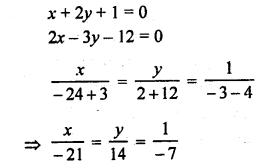Question 2.
Solution: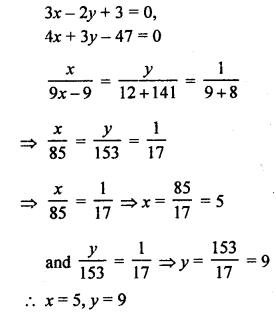Question 3.
Solution:Question 4.
Solution: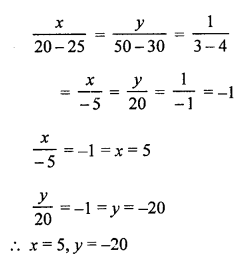Question 5.
Solution:Question 6.
Solution: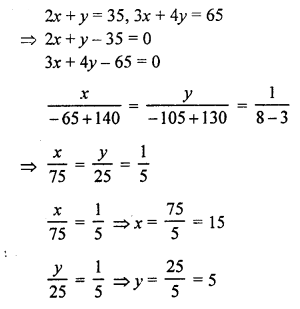x = 15, y= 5

Question 7.
Solution:Question 8.
Solution:Question 9.
Solution: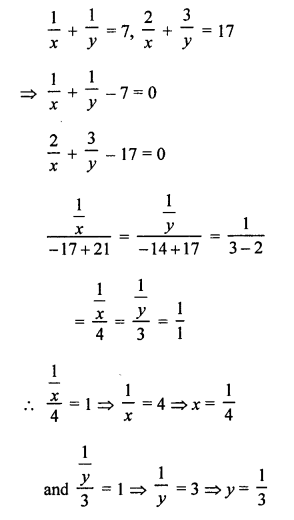Question 10.
Solution: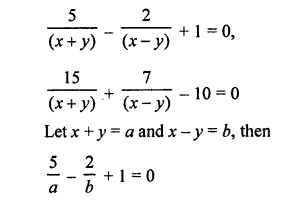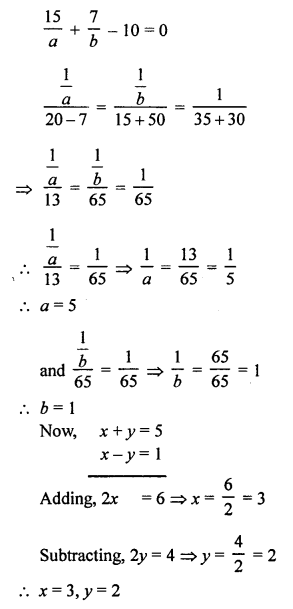Question 11.
Solution: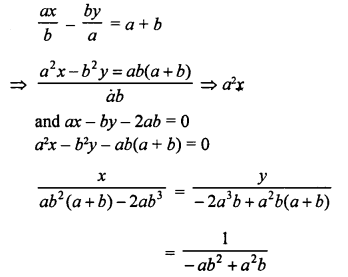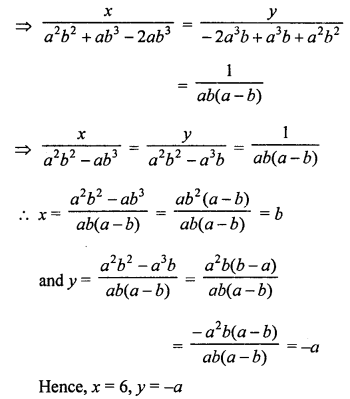Question 12.
Solution: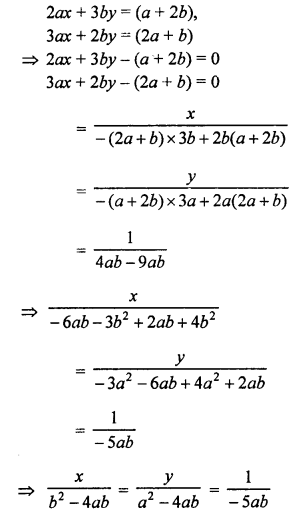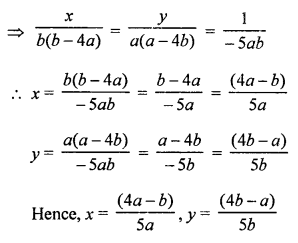Question 13.
Solution:Hope given RS Aggarwal Solutions Class 10 Chapter 3 Linear equations in two variables Ex 3C are helpful to complete your math homework.

If you have any doubts, please comment below. Learn Insta try to provide online math tutoring for you.